36-24 Spoking by Damon Rinard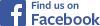Tweet Follow @sheldonbrowncom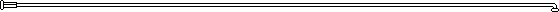## Usefulness of Mismatched Spoke Hole Counts

Whenever the spoke count for the hub doesn't match the one for the rim, you'll need to calculate spoke lengths as this article describes. That may merely be an issue of what hub and rim you have on hand. But also, a mismatched hub and rim can solve a serious problem with a very large hub, such as an electric-bicycle hub motor: excessive spoke angle at the rim. That can result in spoke breakage. Another article on this site shows several ways to use mismatched spoke counts to solve that problem.

## 24 Spokes in a 36 Hole Hub

I've built a few rear wheels using 24 hole rims and 24 spokes in 36 hole hubs. I used a spoke length calculator to find the correct spoke lengths.

For the left side, radial spoke lengths are correct. I laced the left flange in a pattern of one spoke, one empty hole, three spokes, one empty hole, etc. to eliminate torque between left and right flanges. To see a photo of this wheel with the left spokes oriented differently, click on 36_24_2.jpg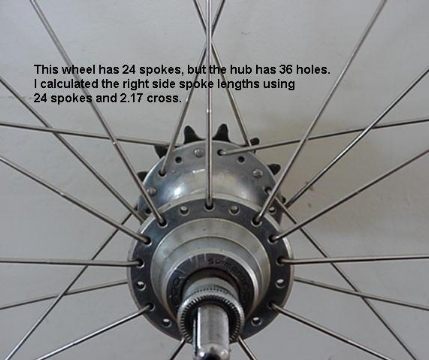For the right side, I entered the number of spokes in the rim (24), and corrected for the different number of holes in the hub by using a decimal number (2.17) for cross. Not many spoke length calculators let you enter a decimal number for cross; I wrote Spocalc.xls in order to do it. Of course, you could just add a millimeter or two to the spoke length, but naturally I wanted to calculate it exactly :-).

This page is about how I calculated the decimal number to enter for cross. Learning what cross really means is a helpful concept first: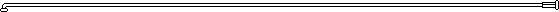## What does Cross Mean Physically?

Imagine the spokes swivel in the rim and their positions are changeable at the hub. Notice that in wheels with the same number of spokes and holes, switching a spoke from one hole in the hub to the next corresponds to an integer increase in cross.

To illustrate this concept, the sketches below show just the right flange's holes and spokes. The leftmost sketch shows 0 cross, or radial spoking. Following along to the right, you can see that moving the spoke to the next hole in the flange increases the number of crosses by one: from radial to 1 cross, then 2 cross, then 3 cross as you shift each spoke by one hole in the flange.Recognizing how this works lets you calculate fractional crosses when using a hub with an excess number of holes.## Excess Number of Hub Holes

When the hub has more holes than you plan to use, then the correct cross number you enter into the spoke length calculator is generally not an integer: it is a decimal number. To find out the decimal cross number to use, follow along below.Sketch Your 36 Hole Hub Flange Draw one flange of the hub you want to use. In this example, it is a 36 hole hub, so the sketch shows 18 holes. Arrange the holes so a vertical line passes between two holes. Choose a characteristic hole you will use for your pattern. In this example, it is the third hole from the top (counting clockwise).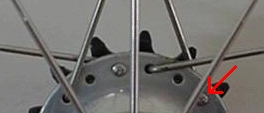Calculate the angle from the vertical to this hole, taken about the axle centerline. This is the angle to the spoke hole you will really use. In this example it is 50 degrees. Call this angle A.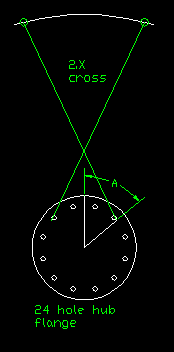Sketch a 24 Hole Hub Flange Now draw one flange of an imaginary hub with the number of holes equal to the number of spokes you are using. In this example, I am using 24 spokes so I sketched a 24 hole hub flange. The sketch shows just one flange, or 12 holes. Arrange the holes so a vertical line passes between two holes. Transfer your angle A to this sketch. Notice that it falls between the second and third spoke holes. Since each hole represents an integer increment in cross, the spot where your spoke falls is a fractional distance between two integer cross values, namely, between 2 cross and 3 cross. Therefore the cross number you should enter into the spoke calculator will be between 2 and 3. But how far past 2 towards 3? Next you must calculate how far, as a fraction of hole spacing, angle A falls between holes 2 and 3.Define the Fraction Calculate the angle from the vertical to the hole just before angle A, measuring clockwise about the axle centerline. Call it angle B. The spoke hole to which angle B is measured represents an integer cross number. In this example angle B is measured to the second hole from the top, so it represents 2 cross. In this example, angle B is 45 degrees. Notice that angle A is larger than angle B (50 > 45). Since B represents 2 cross, you will be adding the fractional amount to 2 cross to get the desired decimal cross number. In other words, Cross number = 2+X , where X represents the fractional increase in cross number. Next you must find the fraction X. Let the fraction X = C/D, where C is the numerator and D is the denominator.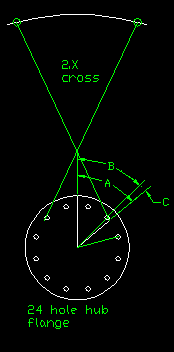Find the Numerator To find the numerator C of the fraction X, Subtract B from A to get a new angle C. In other words, C = A - B. In this example, C = 50 - 45 = 5 degrees.Find the Denominator To find the denominator D of the fraction X, calculate the angular distance from one spoke hole to the next in the imaginary hub's flange. Call this angle D. One way to find D is to divide 360 degrees by the number of holes in one flange. In this example, D = 360/12 = 30 degrees.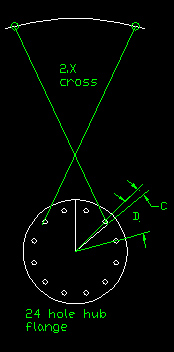Find the Fraction and Decimal Cross Number Since what we want is the additional angle of our spoke location as a fraction of a the angle between two adjacent spoke holes, finding X is simply a matter of dividing C by D, thus: X = C/D In this example, X = C/D = 5/30 = 0.17. Substitute this value of X into the equation to get the cross number, as below: Cross number = 2 + X In this example the cross number is 2 + X = 2 + 0.17 = 2.17.

The decimal cross number (2.17 in this example) is the one you enter into the spoke calculator. It is the only adjustment needed to account for the 36 hole hub in this otherwise 24 spoke wheel. Enter all the other numbers as usual (24 spokes, correct ERD and hub dimensions, etc.) and you'll get the right spoke length.